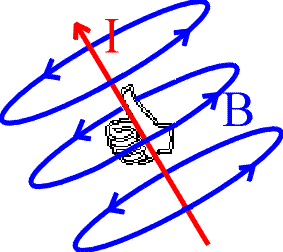Magnetic field of a long wire

Magnetic fields arise from charges, like electric fields; but they are different in that the charges must be moving.

A long straight wire carrying a current is the simplest example of moving charge generating a magnetic field. The magnetic field lines form circles around the wire. The strength of the field depends only on the current I and the distance from the wire r and is given by

B = m0 I / (2 p r)

The direction of the field is such that the magnetic field lines make circles around the wire. To tell which of the two possible directions around the circles that B points in, you use the "curly" right-hand rule: place your right thumb alongside the wire pointing in the direction of the current and your fingers will curl in the direction of the field.The constant m0 = 4 p x 10 -7 is called the magnetic permeability of vacuum. [The fact that it is an exact constant is tied up with the definition of the unit of charge (Coulomb).]

Examples       Magnetic field sources index        Lecture index